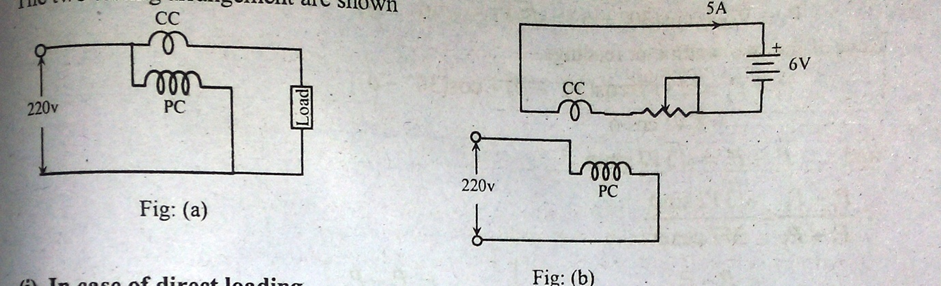Question:
Published on: 23 June, 2022A test with actual loading arrangements would involve a considerable waste of power when the current rating of a meter under test is high. In order to avoid this phantom loading is done. Phantom loading consists of supplying the pressure circuit from a circuit of required normal voltage and the current circuit from a separate low voltage supply. It is possible to circulate the rated current through the current circuit with a low voltage supply as the impedance of this circuit is very low. With this arrangement the total power supplied for the test is that due to the small pressure coil current at normal voltage plus that due to the current circuit current supplied at low voltage. The total power therefore required for testing the meter with phantom loading is comparatively very small. This point is illustration by the following example.

Power consumed in pressure circuit = (200)2/8800 = 5.5W

Power consumed in current circuit = 220*5 = 1100 W

Total power consumed = (5.5+1100) = 1105.5 W